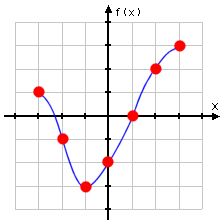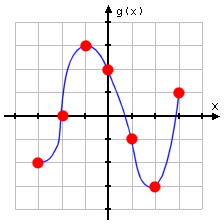Search

Composition of Functions:
Composing with Sets of Points
(page 1 of 6)

Sections: Composing functions that are sets of point, Composing functions at points, Composing functions with other functions, Word problems using composition, Inverse functions and compositionUntil now, given a function  f(x), you would plug a number or another variable in for x. You could even get fancy and plug in an entire expression for x. For example, given  f(x) = 2x + 3, you could find f(y2  1) by plugging y2  1 in for x to get f(y2  1) = 2(y2  1) + 3 = 2y2  2 + 3 = 2y2 + 1.

In function composition, you're plugging entire functions in for the x. In other words, you're always getting "fancy". But let's start simple. Instead of dealing with functions as formulas, let's deal with functions as sets of (x, y) points:

• Let f = {(2, 3), (1, 1), (0, 0), (1, 1), (2, 3)} and
let
g = {(3, 1), (1, 2), (0, 2), (2, 2), (3, 1)}.

Find
(i) f (1), (ii) g(1), and (iii) (g o f )(1).

(i) This type of  exercise is meant to emphasize that the (x, y) points are really (x, f (x)) points. To find  f (1), I need to find the (x, y) point in the set of (x, f (x)) points that has a first coordinate of x = 1. Then f (1) is the y-value of that point. In this case, the point with x = 1 is (1, 1), so:

Advertisement

f (1) = 1

(ii) The point in the g(x) set of point with x = 1 is the point (1, 2), so:

g(1) = 2

(iii) What is "(g o f )(1)"? This is read as "g-compose-f of 1", and means "plug 1 into f, evaluate, and then plug the result into g". The computation can feel a lot easier if I use the following, more intuitive, formatting:

(g o f )(1) = g( f(1))

Now I'll work in steps, keeping in mind that, while I may be used to doing things from the left to the right (because that's how we read), composition works from the right to the left (or, if you prefer, from the inside out). So I'll start with the x = 1. I am plugging this into f(x), so I look in the set of f(x) points for a point with x = 1. The point is (1, 1). This tells me that f(1) = 1, so now I have:   Copyright © Elizabeth Stapel 2002-2011 All Rights Reserved

(g o f )(1) = g( f(1)) = g(1)

Working from the right back toward the left, I am now plugging x = 1 (from "f(1) = 1") into g(x), so I look in the set of g(x) points for a point with x = 1. That point is (1, 2). This tells me that g(1) = 2, so now I have my answer:

(g o f )(1) = g( f(1)) = g(1) = 2

Note that they never told us what were the formulas, if any, for f(x) or g(x); we were only given a list of points. But this list was sufficient for answering the question, as long as we keep track of our x- and y-values.

• Let f = {(2, 3), (1, 1), (0, 0), (1, 1), (2, 3)} and
let
g = {(3, 1), (1, 2), (0, 2), (2, 2), (3, 1)}.

Find (
i) ( f o g)(0), (ii)
( f o g)(1), and (iii) (g o f )(1).
• (i) To find ( f o g)(0), ("f-compose- g of zero"), I'll rewrite the expression as:

( f o g)(0) = f(g(0))

This tells me that I'm going to plug zero into g(x), simplify, and then plug the result into f(x). Looking at the list of g(x) points, I find (0, 2), so g(0) = 2, and I need now to find f(2). Looking at the list of f(x) points, I find (2, 3), so f(2) = 3. Then:

( f o g)(0) = f(g(0)) = f(2) = 3

(ii) The second part works the same way:

( f o g)(1) = f(g(1)) = f(2) = 3

(iii) I can rewrite the composition as (g o f )(1) = g( f(1)) = g(1).

Uh-oh; there is no g(x) point with x = 1, so it is nonsense to try to find the value of g(1). In math-speak, g(1) is "not defined"; that is, it is nonsense.Then (g o f )(1) is also nonsense, so the answer is:

(g o f )(1) is undefined.

Part (iii) of the above example points out an important consideration regarding domains and ranges. It may be that your composed function (the result you get after composing two other functions) will have a restricted domain, or at least a domain that is more restricted than you might otherwise have expected. This will be more important when we deal with composing functions symbolically later.

Another exercise of this type gives you two graphs, rather than two sets of points, and has you read the points (the function values) from these graphs.

• Given f(x) and g(x) as shown below, find ( f o g)(1).

 f(x): g(x):In this case, I will read the points from the graph. I've been asked to find ( f o g)(1) = f(g(1)). This means that I first need to find g(1). So I look on the graph of g(x), and find x = 1. Tracing up from x = 1 to the graph of g(x), I arrive at y = 3. Then the point (1, 3) is on the graph of g(x), and g(1) = 3.

Now I plug this value, x = 3, into f(x). To do this, I look at the graph of f(x) and find x = 3. Tracing up from x = 3 to the graph of  f(x), I arrive at y = 3. Then the point (3, 3) is on the graph of  f(x), and f(3) = 3.

Then ( f o g)(1) = f(g(1)) = f(3) = 3.

• Given f(x) and g(x) as shown in the graphs below, find ( g o f )(x) for integral values of x on the interval 3 < x < 3.

 f(x): g(x):This is asking me for all the values of (g o f )(x) = g( f(x)) for x = 3, 2, 1, 0, 1, 2, and 3.  So I'll just follow the points on the graphs and compute all the values:

(g o f )(3) = g( f(3)) = g(1) = 1

I got this answer by looking at x = 3 on the f(x) graph, finding the corresponding y-value of 1 on the f(x) graph, and using this answer as my new x-value on the g(x) graph. That is, I looked at x = 3 on the f(x) graph, found that this led to y = 1, went to x = 1 on the g(x) graph, and found that this led to y = 1. Similarly:

(g o f )(2) = g( f(2)) = g(1) = 3
(g o f )(1) = g( f(1)) = g(3) = 2
(g o f )(0) = g( f(0)) = g(2) = 0
(g o f )(1) = g( f(1)) = g(0) = 2
(g o f )(2) = g( f(2)) = g(2) = 3
(g o f )(3) = g( f(3)) = g(3) = 1

You aren't generally given functions as sets of points or as graphs, however. Generally, you have formulas for your functions. So let's see what composition looks like in that case...

Top  |  1 | 2 | 3 | 4 | 5 | 6  |  Return to Index  Next >>

 Cite this article as: Stapel, Elizabeth. "Composing with Sets of Points." Purplemath. Available from     https://www.purplemath.com/modules/fcncomp.htm. Accessed [Date] [Month] 2016

Study Skills Survey

Tutoring from Purplemath
Find a local math tutor

 Copyright © 2021  Elizabeth Stapel   |   About   |   Terms of Use   |   Linking   |   Site Licensing Contact Us